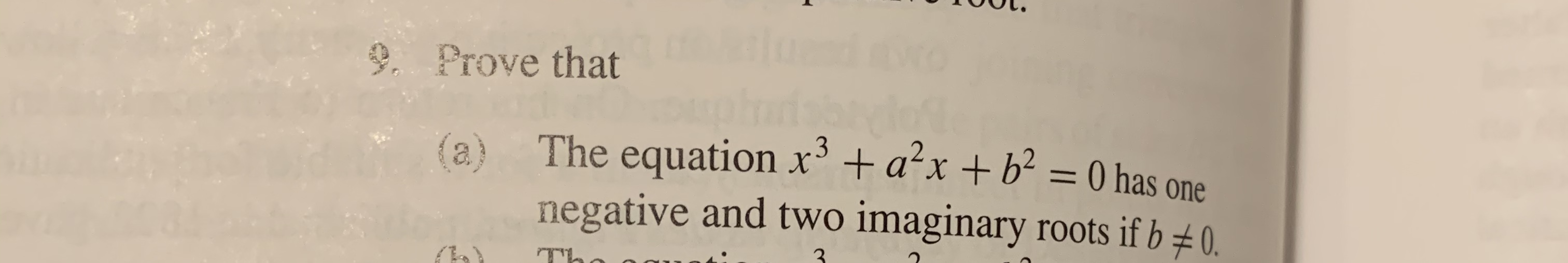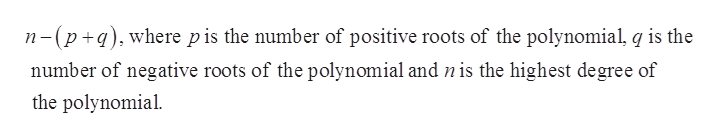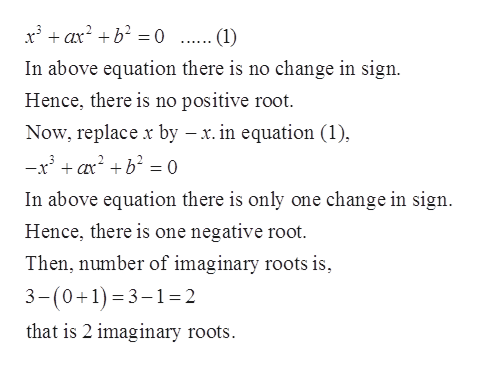# 9. Prove that0 hasThe equation x+ ax+ b2one(a)negative and two imaginary roots if b 0.TL

Question
22 views

#9a using Descartes's rule of signhelp_outlineImage Transcriptionclose9. Prove that 0 has The equation x+ ax+ b2 one (a) negative and two imaginary roots if b 0. TL fullscreen
check_circle

Step 1

Given equation is,

Step 2

Descartes Rule for positive roots: The number of positive real zeroes in a polynomial function  f (x) is the same or less than by an even number as the number of changes in the sign of the coefficients.

Descartes Rule for negative roots: The number of negative real roots is the number of sign changes after putting (– x) in place of x in f (x), or fewer than it by an even number.

Descartes Rule for imaginary roots: The number of imaginary roots is,help_outlineImage Transcriptionclosen-(p q)where pis the number of positive roots of the polynomial, q is the number of negative roots of the polynomial and nis the highest degree of the polynomial fullscreen
Step 3

Consider, b which is not equ...help_outlineImage Transcriptionclosexax2b0 . (1) In above equation there is no change in sign Hence, there is no positive root. Now, replace x by - x.in equation (1) -x3 axb 0 In above equation there is only one change in sign Hence, there is one negative root. Then, number of imaginary roots is, 3-(0+1) 3-1= 2 that is 2 imaginary roots fullscreen

### Want to see the full answer?

See Solution

#### Want to see this answer and more?

Solutions are written by subject experts who are available 24/7. Questions are typically answered within 1 hour.*

See Solution
*Response times may vary by subject and question.
Tagged in

### Math